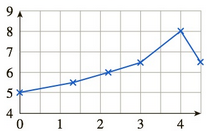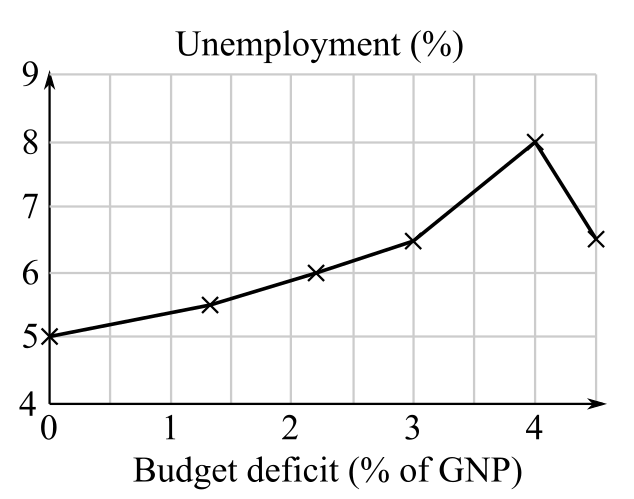Chapter 10.4, Problem 11EFinite Mathematics and Applied Cal...

7th Edition
Stefan Waner + 1 other
ISBN: 9781337274203

Solutions

Chapter
SectionFinite Mathematics and Applied Cal...

7th Edition
Stefan Waner + 1 other
ISBN: 9781337274203
Textbook Problem

In Exercises 1–18, calculate the average rate of change of the given function over the given interval. Where appropriate, specify the units of measurement. [HINT: See Example 1.]Interval: [ 0 , 4 ] Unemployment (%)Budget deficit (% of GNP)

To determine

To calculate: The average rate of change of the provided function over the provided interval [0,4] with the help of the graph provided.Explanation

Given Information:

The interval is [0,4] and the data graph is shown below:

Formula used:

Average rate of change of f(x) over the interval [a,b] is:

Average rate of change of f=Change in fChange in x=ΔfΔx=f(b)f(a)ba

Calculation:

Consider the interval [0,4],

From the graph shown below, find the value of unemployment function U(x) corresponding to interval [0,4]:

Still sussing out bartleby?

Check out a sample textbook solution.

See a sample solution

The Solution to Your Study Problems

Bartleby provides explanations to thousands of textbook problems written by our experts, many with advanced degrees!

Get Started

Use the guidelines of Section 4.5 to sketch the curve. y=(x1)3x2

Single Variable Calculus: Early Transcendentals, Volume I

In Exercises 914, evaluate the expression. 9. (94)3/2

Applied Calculus for the Managerial, Life, and Social Sciences: A Brief Approach

Using for |x| < 1 and differentiation, find a power series for .

Study Guide for Stewart's Single Variable Calculus: Early Transcendentals, 8th

Find for y defined implicity by .

Study Guide for Stewart's Multivariable Calculus, 8th